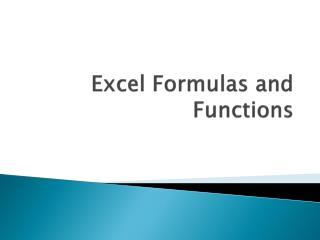DownloadDownload PresentationExcel Formulas and Functions

# Excel Formulas and Functions

Download Presentation## Excel Formulas and Functions

- - - - - - - - - - - - - - - - - - - - - - - - - - - E N D - - - - - - - - - - - - - - - - - - - - - - - - - - -
##### Presentation Transcript

1. Excel Formulas and Functions

2. What is a formula in Excel?

3. A formula is statement written by the user to be calculated. Formulas can be as simple or as complex as the user wants. A formula can contain values, references to cells, defined names, and functions.

4. What is a Formula? • It is a mathematical equation used to caluculate a value. • In Excel formulas must begin with an equal ( = ) sign.

5. Examples of Mathematical Operators • Operator Performs • + (plus sign) = Addition • - (minus sign) = Subtraction • * (asterisk) = Multiplication • / = (slash) Division

6. Examples of Mathematical Operators • ( ) (parentheses) = Controls the order of mathematical operations; calculations within parentheses are performed first • % (percent) = Converts a number into a percentage; for example, when you type 10%, Excel reads the value as .10 • ^ (caret) Exponentiation; for example, when you type 2^3, Excel reads the value as 2*2*2

7. Enter Formulas • 1. Select the cell into which you want to enter the formula. • 2. Type an equal sign (=) to begin the formula. • 3. Enter the first cell referenced in the formula. • 4. Enter the first mathematical operator. • 5. Enter the next cell referenced in the formula. • 6. Continue entering cell references and mathematical operators as needed. • 7. When you have finished creating the formula, press [Enter].

8. Examples of Formulas • = A1+A2+A3+A4 – will add all the values in the four cells • = (8*7)+2 is 58 • = 8*(7+2) is 72 • = 2^2 is 8

9. What is a Function in Excel ?

10. What is a function? • A function is a preset formula in Excel. Like formulas, functions begin with the equal sign ( = ) followed by the function's name and its arguments. The function name tells Excel what calculation to perform. The arguments are contained inside round brackets.

11. What is a Function in Excel? • A function is a piece of code designed to calculate specific values and are used inside formulas.

12. What are Functions? • Functions are typed alongside parenthesizes, where in the arguments if any are listed in between. To use functions in a formula, for example :=COS(3.14) will return the calculated cosine. =NOW() returns the current time. =SUM(1+2+3) *2 will multiply the sum by 2

13. Some Functions • TheSUM, AVERAGE, MAX, MIN, and COUNT functions are entered with the same syntax, including beginning the function with an equal sign (=) and then typing the name of the function and an open parenthesis. You then enter the cell range by dragging to select the cells or by typing the first and last cells in the range. These functions are defined in the following table:

14. Functions

15. Most common used Functions • The SUM function in Excel is specifically designed to add values from different ranges. The SUM Function can be typed into a cell in Excel, or inserted via the Insert Function tool (fx) to the left of your Formula bar. The syntax of the SUM Function is SUM(number1,number2, ...).  SUM is the function name, and contained within the brackets are "arguments", or the pieces of information that Excel requires to complete the Function.  The SUM function allows from 1 to 30 arguments (number 1, number ....) for which you require the total value or SUM.

16. Using Ctrl to Mark Cells • If you wish to add cells that are non-contiguous (not joined together), type in your function =SUM( click in the first cell you wish to add.  Hold down your Ctrl key and click in all other cells you wish to add up, then type in a ).  Typing in a comma instead of selecting with your Ctrl key also works just as efficiently as well.

17. Using SUM to Add a Range from a Different Worksheet • You can easily use SUM to add up the same range in different worksheets.  Click in the cell you want the result of your addition in, then holding down the Shift key, click on the next worksheet that you wish to include in your calculation and highlight the range to be used, then click Enter.

18. Excel’s AutoSum Function • Because adding numbers is probably the most common function that Excel is used for, Excel has a built-in Feature called AutoSum located on the Standard toolbar.  AutoSum is represented as the Greek Capital letter Sigma Σ found under the home tab editing group.  You can use AutoSum to sum a range of cells.  A Range can be one single cell, or many cells.  You can sum cells in a contiguous (no gaps) range of cells, or a non-contiguous (cells not joined together) range.

19. AutoSum • To use AutoSum  you must click in the cell that you wish your result, or addition to appear in.  As a default, AutoSum looks up a column for figures immediately above it to add together. • This works great, unless it encounters a blank row or text.  If it does, then it stops at the last cell with a number in it.  If there are no numbers above it, AutoSum will automatically go to the left looking for numbers to add up, but will again stop at a blank column or text.